Published on

# Lets Talk Data Structures | DSA Series | Part 2 of 3 | Blog Post 2

Author
•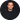Name
Robert Kirby
Title
Lead Full Stack Developer at Y-Squared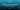## LET’S TALK DATA STRUCTURES

#### Data Structures and Algorithms Series | Part 2 of 3 | Blog Post 2

1- Introduction

2 - Arrays

• 2.1 Introduction
• 2.1 Array Time Complexity
• 2.3 Common Array Methods
• 2.4 Static vs Dynamic Arrays

3 - Hash Tables

• 3.1 Introductions
• 3.2 Hash Functions
• 3.3 Hashtable Time Complexity
• 3.4 Collisons

• 4.1 Introductions
• 4.4 Comparison between the two

5- Stacks and Queues

• 5.1 introduction
• 5.2 Stacks
• 5.3 Queues

6 - Graphs and Trees

• 6.1 Introduction to Graphs and Trees
• 6.2 Trees in detail
• 6.3 Tree Example: Binary Tree(s)
• 6.4 Tree Example: Trie

## 1 - Introduction

The data structures we will be covering In this post are non-primitive data types. Although we won't be covering every data structure and their subsets, we will be covering the important ones and this will give us a good base to work with before part 3 of this series on algorithms! We will also be mentioning some Big O notation along the way to keep up with what we learned in part 1.

There are two types of Non-Primitive Data Types:linear data types, data elements that are arranged sequentially or linearly and non-linear data types, data elements that are not placed sequentially or linearly. In this post we will be looking at examples of both.

Linear data types in this post:Array, Queue, Stack, Linked List, Hash tables*

Non-Linear data types in this post: Trees, Graphs, Hash tables*

Notes:

• Hash tables can be implemented as a linear or non-linear data structure.
• We will not be covering primitive data types in this post (Primitive data type - Wikipedia****)
• The coding examples are written in Javascript, but the general concepts remain language independent.
• I will reference when things are Javascript specific, I will also reference other languages when talking about certain concepts to help differentiate anything that may be confusing.

## 2 - Arrays(Linear Data Type)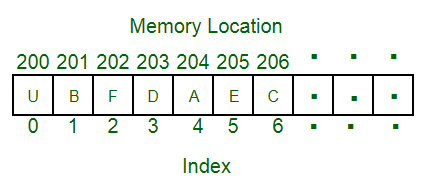#### 2.1 Introduction

Arrays are linear data structures, they are sometimes called lists and are organized pieces of data that have Indexes to identify each value starting from 0, they are also held sequentially(in order) in memory see chart above.

Key point:Arrays in javascript are just objects with integer based keys that act as indexes.

#### 2.2 Array Time Complexity:

• lookup O(1) - constant time
• push O(1) - constant time
• Insert O(n) - linear time
• delete O(n) - linear time

#### 2.3 Common Array Methods:

``````// Initializing the array and storing the info
let exampleArray = ['a', 'b', 'c', 'd']
console.log(`grab example: \${exampleArray}`)  // grabbing info - the third item from the array

// push - add something at the end of the array
exampleArray.push('e')  // O(1) - We are not looping it knows where the last item is
console.log(`push example: \${exampleArray}`)

// pop - remove the last item at the end of the array
exampleArray.pop()  // O(1) - We are not looping it knows where the last item is
console.log(`pop example: \${exampleArray}`)

// unshift - in JS we use unshift add item to the start of the array
exampleArray.unshift('x')
/* O(n) - because we are adding x into the array at the beginning we are moving all the items in the array up one index, so we end up iterating through through the whole array */
console.log(`unshift example: \${exampleArray}`)

// splice - add something in the middle of the array.
/* first argument is the start number, the second number is the delete count, and the third argument is what you are adding */
exampleArray.splice(1, 0, 'Another Example')
/* O(n) - because we are adding a new value into array we have to iterate through the values, add it to the position and shift all the items after it*/
console.log(`splice example: \${exampleArray}`)
``````

#### 2.4 Static vs Dynamic Arrays

Static arrays are fixed in size, the number of elements are specified ahead of time and allocated in adjacent blocks of memory.

Dynamic arrays allow us to copy and rebuild an array at a new location with more memory if we need it.

In lower level languages like C++, we see static arrays a lot more as we specify before time the size of the array and even the type of data it will hold.

C++ Example:int a // An array of 20 integers

In higher level languages like JavaScript and Python we don't need to do this; they automatically allocate memory for us with the arrays being dynamic by default.

Dynamic arrays in higher level languages do the automatic resizing for use, so why do we still use static arrays at all ? Performance is the main answer; static arrays can be useful when we need to be specific with memory allocation, allowing lower level languages like C and C++ to be very fast.

Note: Big-O of appending a dynamic array - append() O(1) can be O(n) why is this ?

``````let exampleArray = ['a', 'b', 'c', 'd'];
let exampleArray = ['a', 'b', 'c', 'd', 'e'];
``````

If there is empty space in the allocated memory we are simply just add the element to the end of the existing array which is O(1) but if there is no more space in the array because this example is dynamic, under the hood, the whole array is iterated through and copied to a new memory location with more space, This can be costly on the system and is O(n).

Arrays are already built into most languages already! It's not necessary to build our own from scratch, but it can be good exercise to practice with.

Here is a good article located on Hackernoon I recommend if you would like to see how this is done by @vibhorthakral - Vibhor Thakral on building your own array from scratch in JavaScript:

## 3 - Hash Tables( Can be Linear or Non-Linear Data Type))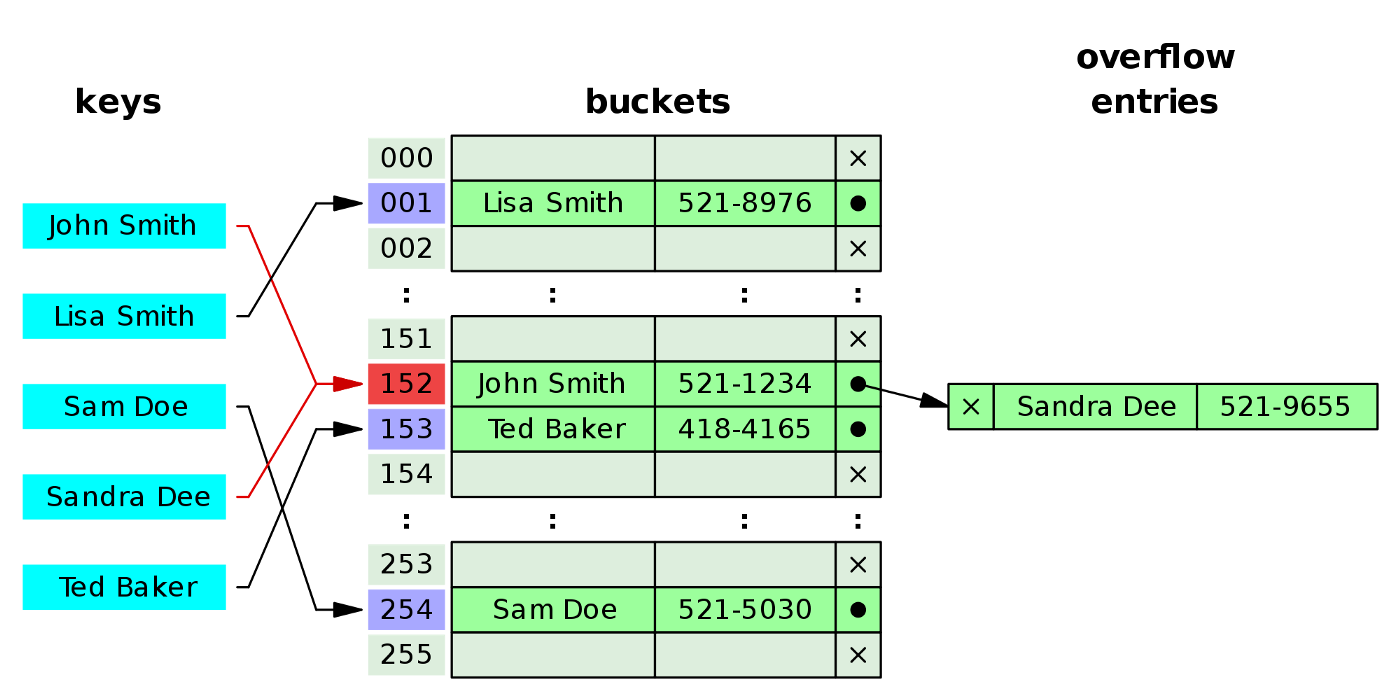#### 3.1 Introduction

Hash tables also known as Hashmaps, Dictionaries, Maps and Objects depending on the language you are working with are an implementation of an associative array, a list of key-value pairs that allow you to retrieve a value via a key. Associative arrays use keys to find the address located in memory, whereas indexed arrays find the value based on their indexed position.

How do we produce this key ? Using a hash function, but we will get back to that in the next section.

In Javascript there are two ways of implementing hash tables: using the Object data type and using the Map Object.

Example Object 1:

``````// stockList.nikeShoes = “10000”
// object - key - value

stockList = {
nikeShoes: “10000”
}
``````

Example Object 2:

``````let testUser = {
name: 'rob',
age: '28',
job: 'Developer'
}

console.log(testUser.name) // Access value using the dot notation and the key.
testUser.favoriteFood = 'pizza'  // We can add a new property and value easily the same way.
console.log(testUser)  If we can check the object in the console we can see it's now added
``````

Note: We access and update the properties of a Javascript object by using the:

• Dot property accessor: object.property
• Square brackets property access: object['property']
• Object destructuring: const { property } = object

Please check out this great article from Dmitri Pavlutin if you would like to know more about these topics.

https://dmitripavlutin.com/access-object-properties-javascript/

3.2 Hash Functions

Internally a hash table utilizes a hash function, it takes the key value and converts it into a number which will be the index/address of the data. This allows us to look up data quickly as we know the memory address location. Most languages handle this under the hood so we don't have to worry about writing one.

Notes:

• Hash functions can be widely seen in cryptography; some examples would be MD5, SHA-1, SHA-256 ect.
• A hash function is one way.
• We call a hash functionidempotent; this means the hash will always be the same for the same input (Providing we are using the same hashing protocol).

#### 3.3 Hashtable Time Complexity

• Insert O(1)
• lookup O(1)
• delete O(1)
• search O(1)

Why are hash tables fast? We know exactly where the location of the data is, it is also not ordered so when delete or add data we don't need to rearrange all the index positions like an array. This doesn't mean that hashtables are perfect as we will find out in the next section.

#### 3.4 Collisons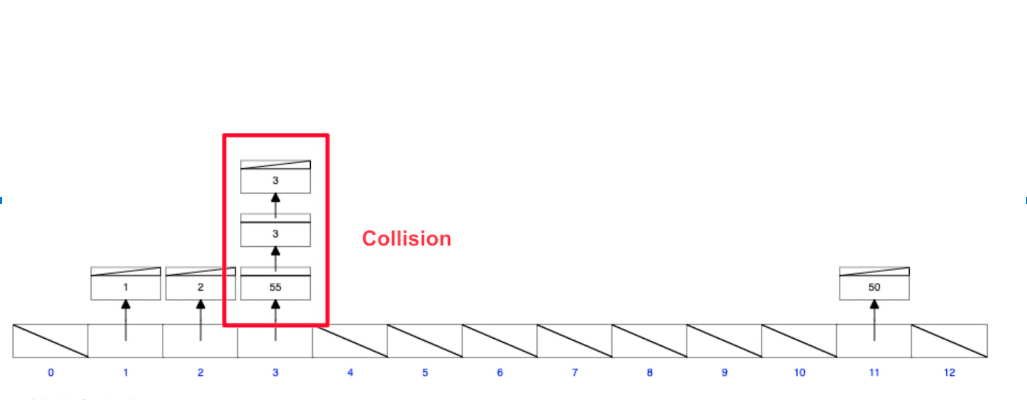Screenshot taken from:https://www.cs.usfca.edu/~galles/visualization/OpenHash.html

A hash collision is when two values get stored in the same index/address position, with enough data and limited memory; this is inevitable and will actually create another data structure called a linked list.

When a linked list is created to combat the collision this is called chaining, the first node will now act as the head entry point of the linked list; we will cover linked lists in detail after this section.

Why does this matter ? This slows down the time complexity as we will now need to iterate through the items at that index address. Big-O is now comparable to an array with linear time O(n).

It’s worth noting in JavaScript the Map object was made to combat some of the pitfalls of hash tables using objects, such as collisions. A key in the Map may only occur once; it is unique in the Map's collection.

## 4 - Linked Lists(Linear Data Type)#### 4.1 Introductions

We briefly mentioned a linked list in the last section when talking about hash table collisions.

What is it ? A linked list is a linear data structure, in which the elements are not stored at contiguous memory locations. Linked lists are made up of nodes, each node that contains a value + pointer(s).

In languages like C and C++ A pointer is a variable that stores the memory address of another variable as its value. In Javascript there are no actual pointers, instead of pointers we use references pointing to an existing variable.

Pointer C Example:

``````int Age = 28;  //  int variable
int* ptr = &age;  // pointer variable, with the name ptr, that stores the address of age

// Output the value of Age (28)
printf("%d\n", age);

// Output the memory address of age (0x12d2ffe5e809)
printf("%p\n", &age);

// Output the memory address of age with the pointer (0x12d2ffe5e809)
printf("%p\n", ptr);
``````

Reference in JavaScript Example:

``````let luckyNumbers = [10,10,10];
let stillMyNumbers = luckyNumbers;
stilMyNumbers.push(0);
console.log(luckyNumbers);  // [10, 10, 10, 0]
console.log(stillMyNumbers);  // [10, 10, 10, 0]
``````

On the surface references are quite easy to comprehend, but in the background references act very differently to pointers for example only compound values (object’s, array’s) can be assigned by reference.

I don't want to spend to long on them in this article butif you have never encountered references before,I recommend you read this great article aboutJavascript References written by Naveen Karippai on Medium:

In the following sections I will use the word pointers when describing the nodes even though the code examples are written in Javascript.

Singly Linked lists contain nodes that have two elements: the value of the data and the pointer which points to the next node in line. The first node is called the head and the last node is called the tail which is always pointing or linking to a null reference, indicating the end of the list.

An advantage a linked list has over an array is when we add a new node to a linked list, it only affects that area where we are removing or adding an item whereas in an array this may require all the indexes to be changed, which costs us in time complexity.

A disadvantage a linked list has is that they are scattered in memory so it's quite slow to search in comparison to an array.

Time complexity:

• prepend O(1) - Add something to the start
• append O(1) - Add something to the end of
• lookup O(n) - Or Traversal to look for an item
• insert O(n) - have to go one by one and find the index so could be O(n)
• delete O(n) - have to also go find it

``````// For the sake of simplicity and so you can easily see what's going on I’ve only added the insert and print methods to the example below.

class NodeSingle {
constructor(value) {
this.value = value
this.next = null
}
}
constructor() {
this.tail = null
this.length = 0
}
insert(val) {
const newNode = new NodeSingle(val)
this.tail = newNode
} else {
this.tail.next = newNode
this.tail = newNode
}
this.length++
return this
}
print() {
while (current) {
console.log(current.value)
current = current.next
}
}
}

``````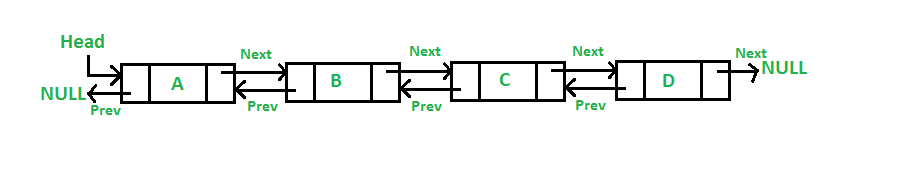Time complexity:

• prepend O(1)
• append O(1)
• insert O(n)
• delete O(n)
• lookup O(n) - Can technically be O(n/2) so more efficient because it can traverse both ways.

Doubly Linked Lists contain an extra piece of data allowing it to point forward and back this means we can also traverse the linked list back to front which has its advantages, the downside is we have to hold some more memory.

All the nodes have next and previous pointers but the head node previous pointer points to null and the tail node next pointer points to null.

``````// For the sake of simplicity and so you can easily see what's going on I’ve only added the insert and print methods to the example below.

class NodeDouble {
constructor(value) {
this.value = value
this.prev = null
this.next = null
}
}
constructor() {
this.tail = null
this.length = 0
}
insert(val) {
const newNode = new NodeDouble(val)
this.tail = newNode
} else {
this.tail.next = newNode
newNode.prev = this.tail
this.tail = newNode
}
this.length++
return this
}
printList() {
console.log(list)
while (current.next) {
console.log(current)
current = current.next
}
console.log(current)
} else {
console.log('empty list')
}
}
print() {
while (current) {
console.log(current.value)
current = current.next
}
}
}

``````

#### 4.4 Comparison between the two?

When should you use a single vs double?

Singly:

• Uses less memory so it's a bit faster.
• It’s better to use singly when you are limited by memory and you want to do fast insersention and deleting.
• On the downside it can't be traversed back to front.
• If we lose the head node it can actually be lost in memory forever.

Doubly:

• It can be traversed from the front and back.
• The downside is it's more complex and uses more memory.
• Less likely to be lost in memory.
• It's good when you don't have memory limitations and is better for searching.

A real life example of a linked list is your computer filesystem or internet history.

The general gist of the circular linked list is a linked list where all nodes are connected to form a circle. In a circular linked list, the first node and the last node are connected to each other which forms a circle. There is no NULL at the end.

## 5 -Stacks and Queues(Linear Data Types)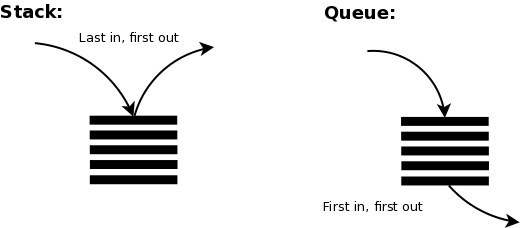Chart source - Stacks and Queues by Isa Weaver:https://medium.com/x-organization/stack-and-queue-60f365963552

Stacks and Queues are very similar; they are both linear data structures and we go through them one by one. They are implemented in a similar way; the main difference is how they get removed.

There are no random access operations in Stacks and Queues and we are usually only concerned with the first or last element. There are less actions and operations available to us which can be an advantage because we have more control.

#### 5.2 Stacks

Think of a stack like a stack of plates where one goes on top of the next one. We use the LIFO method (Last in First Out) with a stack which means when we add a plate to the top it's the first one we take back off.

Stack Overflow otherwise known as our favorite website gets its name from a stack error that occurs when the allocated memory in the stack runs out!

A real life example of stacks in action are the back and forward button on your internet browser; another is the undo and redo functionality.

Common Methods + Time Complexity:

• pop O(1) - remove top item
• push O(1) - add top item
• peek O(1) - view top item
• Lookup O(n) - Not very commonly used*

JavaScript doesn't have its own stack data structure:

• We can build stacks with arrays or linked lists.
• Both will work well, so it's really a preference.
• Arrays allow cache locality which makes them technically faster because the values are beside each other but a linked list has better dynamic memory so we can keep adding more elements without incurring the time and space cost.

#### 5.3 Queues

Queues on the other hand use FIFO method (First in First Out), Think of a roller coaster queue!

Real life examples of queues would be a printer queue, another would be the car ride request queue on Uber for example.

Common Methods + Time Complexity:

• enqueue O(1) - like push but add to queue back of line
• dequeue O(1) - like pop but remove first entry
• peek O(1) - Whos up next
• Lookup O(n) - Not very commonly used*

Javascript also doesn't have its own queue data structure:

• We can build with arrays or linked lists.
• A linked list is better - You'd never want to use an array because of the index changes associated with them.
• With a linked list we just need to change the head node.

## 6 - Trees and Graphs(Non-Linear Data Types)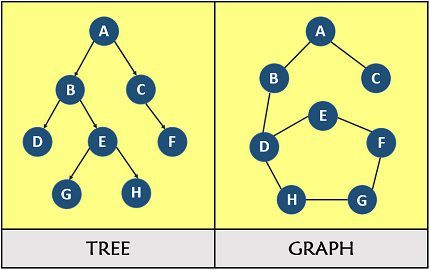#### 6.1 Introduction to Graphs and Trees

Every tree is a graph, but not every graph is a tree.

We will focus more on trees in this section but we will quickly run through graphs and what makes certain graphs trees.

Graph :

A graph is a collection of two sets V and E where V is a finite non-empty set of vertices and E is a finite non-empty set of edges -

• Vertices are nothing but the nodes in the graph.
• Two adjacent vertices are joined by edges.
• Any graph is denoted as G = {V, E}.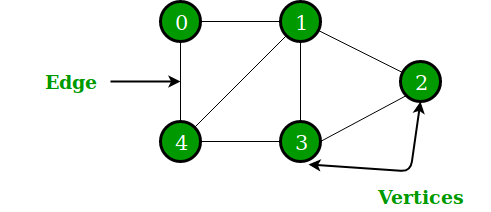Tree:

A tree is a finite set of one or more nodes such that –

• There is a specially designated node called root.
• The remaining nodes are partitioned into n>=0 disjoint sets T1, T2, T3, …, Tn
• where T1, T2, T3, …, Tn are called the subtrees of the root.

The concept of a tree is represented by the following Fig.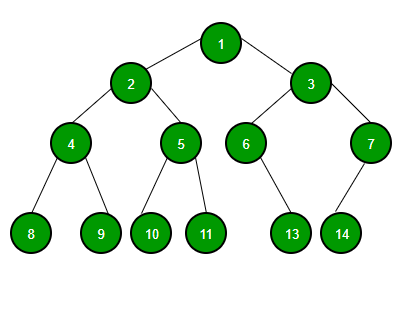- End of sample

Some key differences:

• Trees are always directed, graphs can be directed and undirected.
• Trees always have a root(parent) node, there is no root node in a graph.

#### 6.2 Trees in detail

Trees are a data structure in the graph family, they are hierarchical in order with a Root node (parent) and children, a tree can have zero or more child nodes. Children on the same level are called siblings and leafs are another name for the children nodes at the very ends of trees. This parent child relationship is unidirectional meaning it only goes one way.

Like in linked lists we have nodes that contain information, infact a linked list is technically a type of three but with just one path and its linear, trees have multiple paths.

There are many types of tree data structures, just like in real life there are different types of trees but are similar with minor alterations.

Examples of real life trees you might know:

• The Document Object Model(The DOM) is a tree data structure. It starts with the HTML element which is the root then it has two children the head and the body and so on.
• Abstract Syntax Tree - You may have heard of it but If not its part of the process used by a compiler makes sense of source code. If you don't know about this process here is a easy to understand article on it Abstract Syntax Tree (AST) - Explained in Plain English on dev.to by Bala Priya C.

#### 6.3 Tree Example: Binary Tree(s)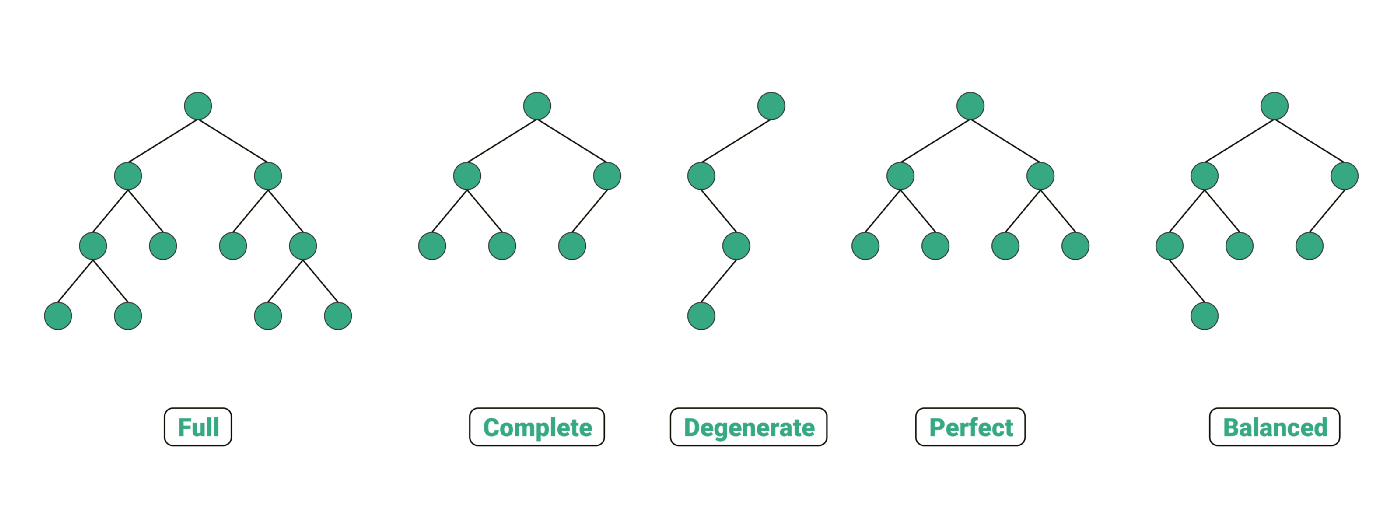Illustration by Anand K Parmar:https://medium.com/towards-data-science/5-types-of-binary-tree-with-cool-illustrations-9b335c430254

Each node can only have 0, 1 or 2 nodes and each child can only have one parent, each node represents a certain state.

Binary tree node example:

``````function BinaryTreeNode(value) {
the.value = value
this.left = null
this.right = null
}
``````

I recommend checking out this article Different Types of Binary Tree with colourful illustrations” - by Anand K Parmar It explains all five of the binary trees in the above diagram quickly and with illustrations.

Notes:

• Long unbalanced binary trees turn into linked lists and become less efficient. We lose our log n performance operations and it just becomes O(n) .
• We can combat this by using AVL Trees and Red Black Trees which are self balancing trees, most languages now have libraries and tools to do this automatically.
• Trees aren't the fastest at anything but there are certain conditions where they outperform arrays and objects.

#### 6.4 Tree Example: Trie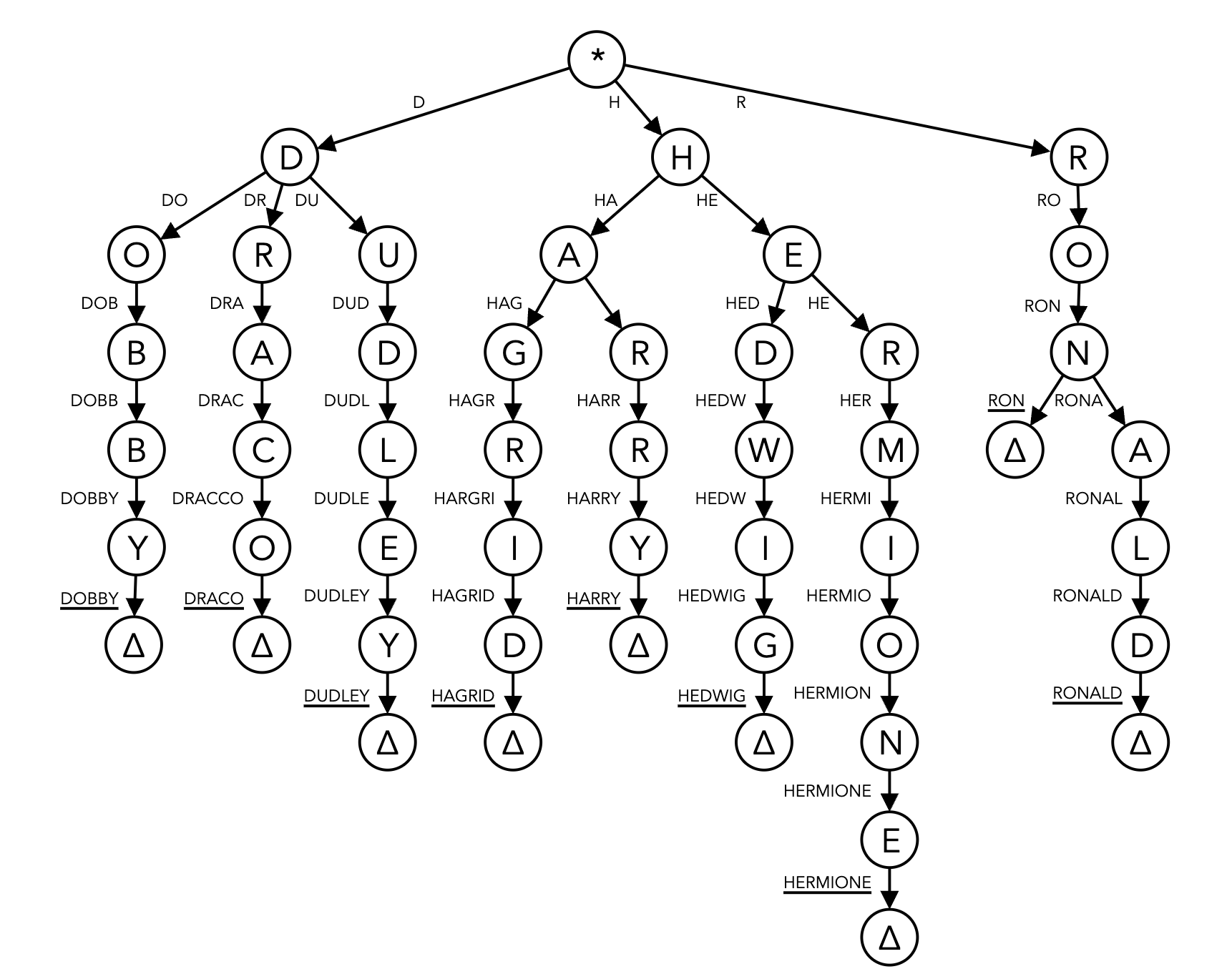Graph sourced from:https://datastructures.maximal.io/tries/

A Trie is a specialized tree for searching:

• It has a starting root node which is empty.
• It's not like a binary tree that can only have two children, a trie can have many children, it can also be called a prefix tree.
• Real world examples of a trie is autocomplete function in google search.
• The big O of a trie is O(length of the word). Tires have excellent space complexity due to the organization of the data.

## 7 - Contact and Links

Please fill out the form on https://y-squared.com/contact or email us at team@y-squared.com.

The Author:

This post was written by Robert from Y-Squared: